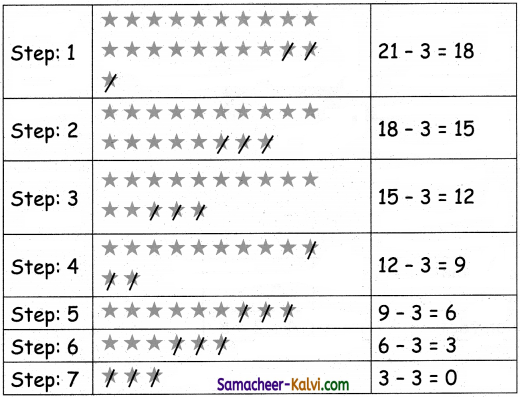## Samacheer Kalvi 3rd Standard Maths Book Solutions Term 3 Chapter 3 Patterns

Iterative patterns and processes:

Question 1.
Continue the pattern to complete the rangoli.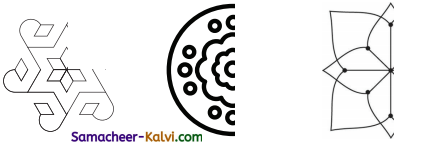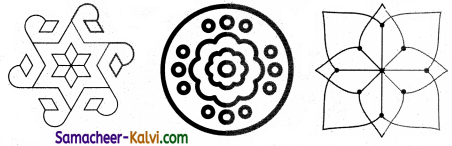Question 2.
Make a pattern of your own on the given category and draw a rangoli.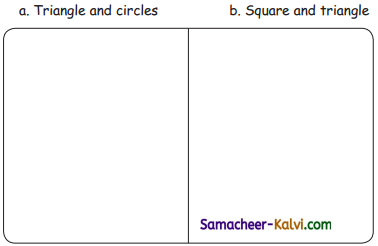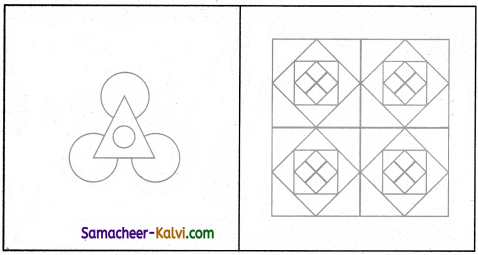Question 3.
Draw a pullikolams by looking at the reference image without lifting your hand.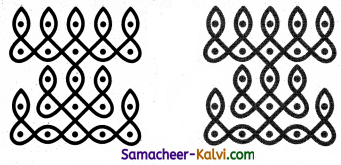Question 4.
Draw 2 pullikolams of your choice along the given dots.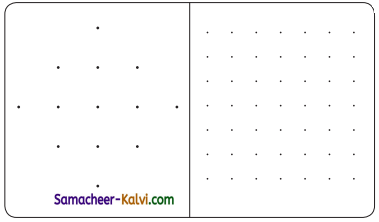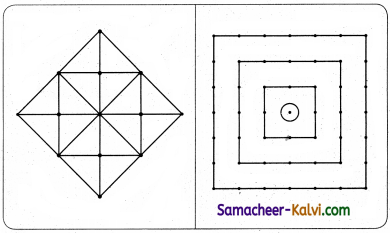Patterns obtained by adding numbers (Text Book Page No. 22):

Complete the addition table and observe the pattern in them.

Question 2.
Write the numbers which add upto the given addition fact.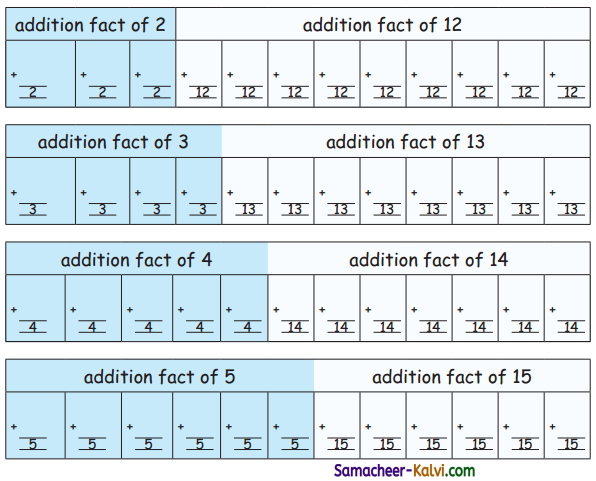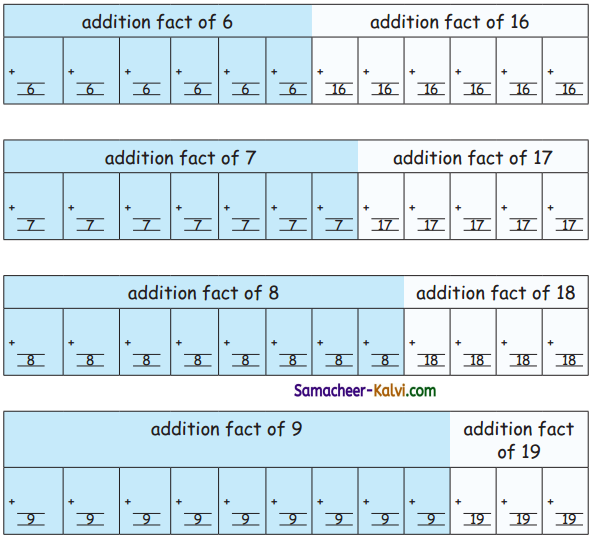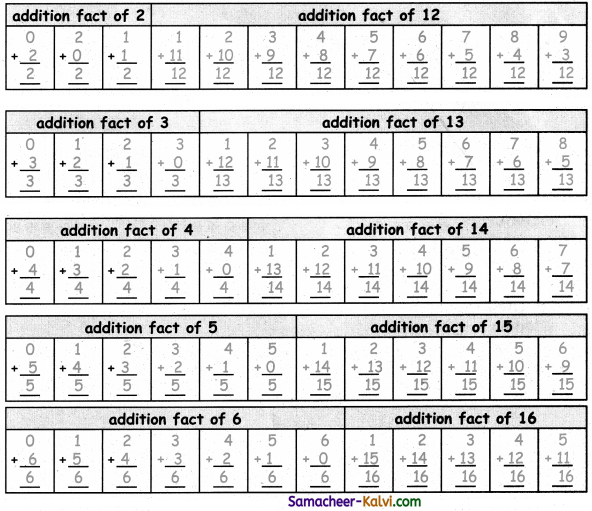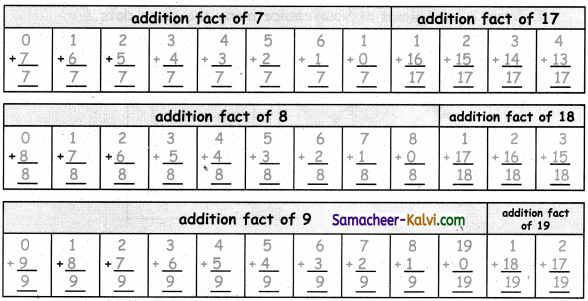Question 3.
Find out the missing numbers and write them in the given blank.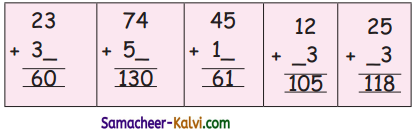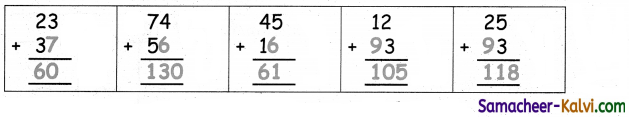Exercise (Text Book Page No. 25):

Continue the patterns by using multiplication as repeated addition.

a.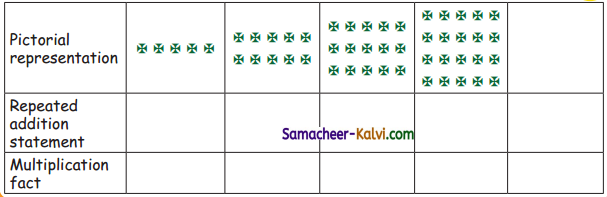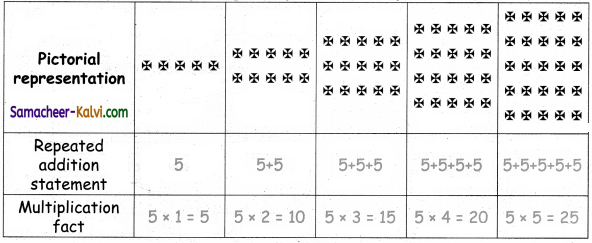b.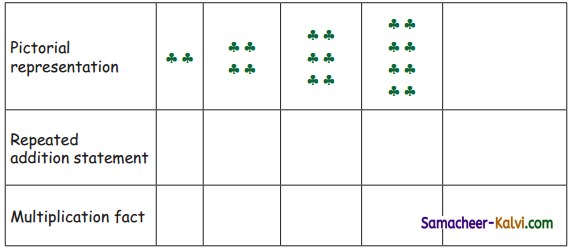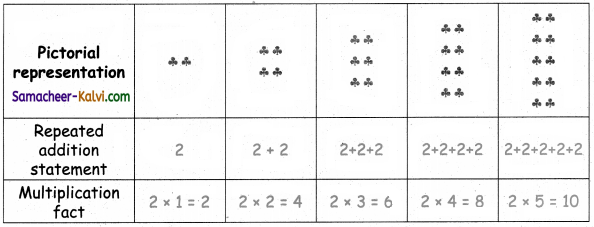Exercise (Text Book Page No. 26):

Express the division facts as repeated subtraction using patterns.

a) 24 ÷ 3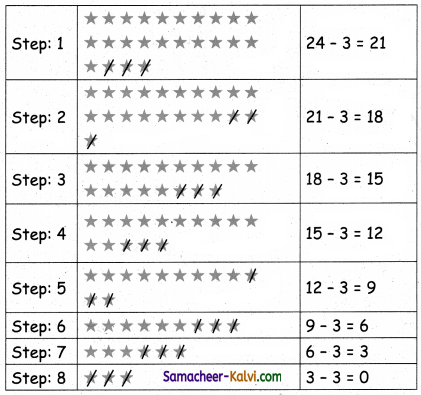24 ÷ 3 = 8b) 22 ÷ 2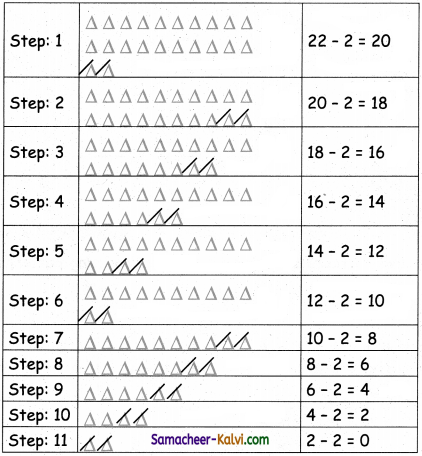22 ÷ 2 = 11c) 32 ÷ 4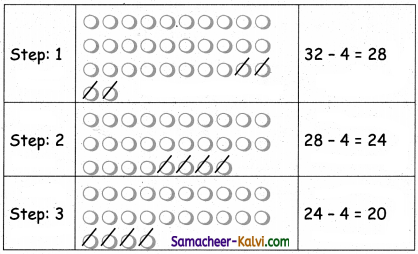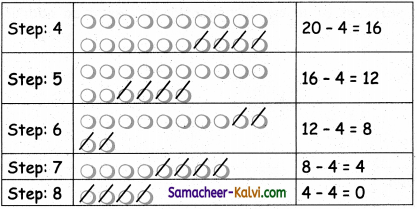32 ÷ 4 = 8d) 15 ÷ 3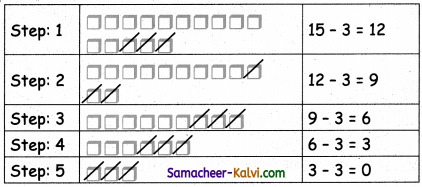15 ÷ 3 = 53rd Standard Maths Guide Patterns Additional Questions and Answers

Question 1.
Find out the missing numbers and write them in the given blank.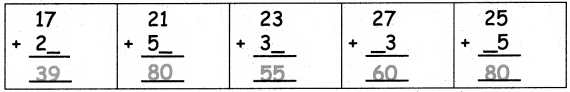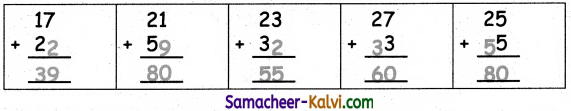Question 2.
Continue the patterns by using multiplication as repeated addition.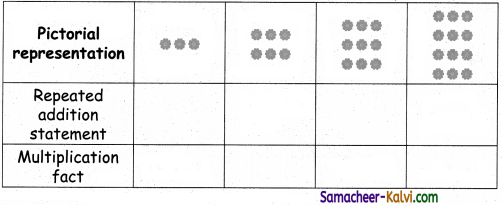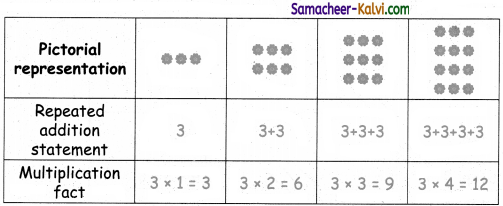Question 3.
Express the division facts as repeated subtraction using patterns.

a) 24 ÷ 4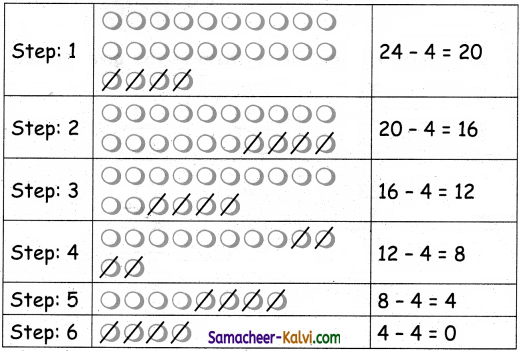24 ÷ 4 = 6b) 21 ÷ 3